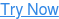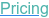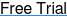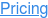#### SOFTWARE#### KNOWLEDGE BASE# What statistical test should I use?

March 2, 2021

What statistical test should I use? It is often said that the design of a study is more important than the analysis. A badly designed study can never be retrieved, whereas a poorly analyzed study can usually be re-analyzed.

# Types of Statistical Tests

Updated: March 2021

In terms of selecting a statistical test, the most important question is "what is the main study hypothesis?". For example, nQuery has a vast list of statistical procedures to calculate sample size, in fact over 1000 sample size scenarios are covered. However, it is important that these are paired with a correctly designed trial.

The correct statistical test to use not only depends on your study design, but also the characteristics of your data. This will be a result of your research questions/hypotheses you are trying to answer.

The graph and table below can be used as a guide for which statistical test or descriptive statistic to use in your research. In order to use it, you must be able to identify all the variables in the data set and tell what kind of variables they are.As you know and can see there's a wide range of statistical tests to choose from.

The decision of which statistical test to use depends on:

1. The research design
2. The distribution of the data
3. The type of variable
When choosing the correct test,  ask yourself the following questions:  What kind of data have you collected? What is your goal?

 Goal Measurement(from Gaussian Population) Rank, Score, or Measurement(from Non- Gaussian Population) Binomial(Two Possible Outcomes) Survival Time Describe one group Mean, SD Median, interquartile range Proportion Kaplan Meier survival curve Compare one group to a hypothetical value One-sample t-test Wilcoxon test Chi-squareor Binomial test ** Compare two unpaired groups Unpaired t test Mann-Whitney test Fisher's test(chi-square for large samples) Log-rank test or Mantel-Haenszel* Compare two paired groups Paired t test Wilcoxon test McNemar's test Conditional proportional hazards regression* Compare three or more unmatched groups One-way ANOVA Kruskal-Wallis test Chi-square test Cox proportional hazard regression** Compare three or more matched groups Repeated-measures ANOVA Friedman test Cochrane Q** Conditional proportional hazards regression** Quantify association between two variables Pearson correlation Spearman correlation Contingency coefficients** Predict value from another measured variable Simple linear regressionor Nonlinear regression Nonparametric regression** Simple logistic regression* Cox proportional hazard regression* Predict value from several measured or binomial variables Multiple linear regression* or Multiple nonlinear regression** Multiple logistic regression* Cox proportional hazard

## What type of statistical test to use?

Below is an extract from the Handbook of Biological Statistics by Prof John H. McDonald.
This can be used as a further guide
to decide what statistical test to use in your research.

Test Nominal Variables Measurement Variables Ranked Variables Purpose Notes Example
Exact test for goodness-of-fit 1 test fit of observed frequencies to expected frequencies use for small sample sizes (less than 1000) count the number of red, pink and white flowers in a genetic cross, test fit to expected 1:2:1 ratio, total sample <1000
Chi-square test of goodness-of-fit 1 test fit of observed frequencies to expected frequencies use for large sample sizes (greater than 1000) count the number of red, pink and white flowers in a genetic cross, test fit to expected 1:2:1 ratio, total sample >1000
G–test of goodness-of-fit 1 test fit of observed frequencies to expected frequencies used for large sample sizes (greater than 1000) count the number of red, pink and white flowers in a genetic cross, test fit to expected 1:2:1 ratio, total sample >1000
Repeated G–tests of goodness-of-fit 2 test fit of observed frequencies to expected frequencies in multiple experiments - count the number of red, pink and white flowers in a genetic cross, test fit to expected 1:2:1 ratio, do multiple crosses

Test Nominal Variables Measurement Variables Ranked Variables Purpose Notes Example
Fisher's exact test 2 test hypothesis that proportions are the same in different groups use for small sample sizes (less than 1000) count the number of live and dead patients after treatment with drug or placebo, test the hypothesis that the proportion of live and dead is the same in the two treatments, total sample <1000
Chi-square test of independence 2 test hypothesis that proportions are the same in different groups use for large sample sizes (greater than 1000) count the number of live and dead patients after treatment with drug or placebo, test the hypothesis that the proportion of live and dead is the same in the two treatments, total sample >1000
G–test of independence 2 test hypothesis that proportions are the same in different groups large sample sizes (greater than 1000) count the number of live and dead patients after treatment with drug or placebo, test the hypothesis that the proportion of live and dead is the same in the two treatments, total sample >1000
Cochran-Mantel-Haenszel test 3 test hypothesis that proportions are the same in repeated pairings of two groups alternate hypothesis is a consistent direction of difference count the number of live and dead patients after treatment with drug or placebo, test the hypothesis that the proportion of live and dead is the same in the two treatments, repeat this experiment at different hospitals

Test Nominal Variables Measurement Variables Ranked Variables Purpose Notes Example
Arithmetic mean 1 description of central tendency of data - -
Median 1 description of central tendency of data more useful than mean for very skewed data median height of trees in forest, if most trees are short seedlings and the mean would be skewed by a few very tall trees
Range 1 description of dispersion of data used more in everyday life than in scientific statistics -
Variance 1 description of dispersion of data forms the basis of many statistical tests; in squared units, so not very understandable -
Standard deviation 1 description of dispersion of data in same units as original data, so more understandable than variance -
Standard error of the mean 1 description of accuracy of an estimate of a mean - -
Confidence interval 1 description of accuracy of an estimate of a mean - -

Test Nominal Variables Measurement Variables Ranked Variables Purpose Notes Example
One-sample t–test 1 test the hypothesis that the mean value of the measurement variable equals a theoretical expectation - blindfold people, ask them to hold arm at 45° angle, see if mean angle is equal to 45°
Two-sample t–test 1 1 test the hypothesis that the mean values of the measurement variable are the same in two groups just another name for one-way anova when there are only two groups compare mean heavy metal content in mussels from Nova Scotia and New Jersey
One-way anova 1 1 test the hypothesis that the mean values of the measurement variable are the same in different groups - compare mean heavy metal content in mussels from Nova Scotia, Maine, Massachusetts, Connecticut, New York and New Jersey
Tukey-Kramer test 1 1 after a significant one-way anova, test for significant differences between all pairs of groups - compare mean heavy metal content in mussels from Nova Scotia vs. Maine, Nova Scotia vs. Massachusetts, Maine vs. Massachusetts, etc.
Bartlett's test 1 1 test the hypothesis that the standard deviation of a measurement variable is the same in different groups usually used to see whether data fit one of the assumptions of an anova

compare standard deviation of heavy metal content in mussels from Nova Scotia, Maine, Massachusetts, Connecticut, New York and New Jersey

Test Nominal Variables Measurement Variables Ranked Variables Purpose Notes Example
Nested anova 2+ 1 test hypothesis that the mean values of the measurement variable are the same in different groups, when each group is divided into subgroups subgroups must be arbitrary (model II) compare mean heavy metal content in mussels from Nova Scotia, Maine, Massachusetts, Connecticut, New York and New Jersey; several mussels from each location, with several metal measurements from each mussel
Two-way anova 2 1 test the hypothesis that different groups, classified two ways, have the same means of the measurement variable - compare cholesterol levels in blood of male vegetarians, female vegetarians, male carnivores, and female carnivores
Paired t–test 2 1 test the hypothesis that the means of the continuous variable are the same in paired data just another name for two-way anova when one nominal variable represents pairs of observations compare the cholesterol level in blood of people before vs. after switching to a vegetarian diet
Wilcoxon signed-rank test 2 1 test the hypothesis that the means of the measurement variable are the same in paired data used when the differences of pairs are severely non-normal compare the cholesterol level in blood of people before vs. after switching to a vegetarian diet, when differences are non-normal

Test Nominal Variables Measurement Variables Ranked Variables Purpose Notes Example
Linear regression 2 see whether variation in an independent variable causes some of the variation in a dependent variable; estimate the value of one unmeasured variable corresponding to a measured variable - measure chirping speed in crickets at different temperatures, test whether variation in temperature causes variation in chirping speed; or use the estimated relationship to estimate temperature from chirping speed when no thermometer is available
Correlation 2 see whether two variables covary - measure salt intake and fat intake in different people's diets, to see if people who eat a lot of fat also eat a lot of salt
Polynomial regression 2 test the hypothesis that an equation with X2, X3, etc. fits the Y variable significantly better than a linear regression - -
Analysis of covariance (ancova) 1 2 test the hypothesis that different groups have the same regression lines first test the homogeneity of slopes; if they are not significantly different, test the homogeneity of the Y-intercepts measure chirping speed vs. temperature in four species of crickets, see if there is significant variation among the species in the slope or Y-intercept of the relationships

Test Nominal Variables Measurement Variables Ranked Variables Purpose Notes Example
Multiple regression 3+ fit an equation relating several X variables to a single Y variable - measure air temperature, humidity, body mass, leg length, see how they relate to chirping speed in crickets
Simple logistic regression 1 1 fit an equation relating an independent measurement variable to the probability of a value of a dependent nominal variable - give different doses of a drug (the measurement variable), record who lives or dies in the next year (the nominal variable)
Multiple logistic regression 1 2+ fit an equation relating more than one independent measurement variable to the probability of a value of a dependent nominal variable - record height, weight, blood pressure, age of multiple people, see who lives or dies in the next year

Test Nominal Variables Measurement Variables Ranked Variables Purpose Notes Example
Sign test 2 1 test randomness of direction of difference in paired data - compare the cholesterol level in blood of people before vs. after switching to a vegetarian diet, only record whether it is higher or lower after the switch
Kruskal–Wallis test 1 1 test the hypothesis that rankings are the same in different groups often used as a non-parametric alternative to one-way anova 40 ears of corn (8 from each of 5 varieties) are ranked for tastiness, and the mean rank is compared among varieties
Spearman rank correlation 2 see whether the ranks of two variables covary often used as a non-parametric alternative to regression or correlation 40 ears of corn are ranked for tastiness and prettiness, see whether prettier corn is also tastier

References
Chapter 37+45 of the second edition of Intuitive Biostatistics Harvey Motulsky.
McDonald, J.H. 2014. Handbook of Biological Statistics (3rd ed.). Sparky House Publishing, Baltimore, Maryland.
Campbell MJ, Machin D. In: Medical Statistics: A Common-sense Approach , 2nd edn. Chichester: Wiley, 1993:2.
Stats Flow Chart

## You May Also Like

These Stories on Guide to Sample Size